# Control PR2 Arm Movements Using ROS Actions and Inverse Kinematics

This example shows how to send commands to robotic manipulators in MATLAB®. Joint position commands are sent via a ROS action client over a ROS network. This example also shows how to calculate joint positions for a desired end-effector position. A rigid body tree defines the robot geometry and joint constraints, which is used with inverse kinematics to get the robot joint positions. You can then send these joint positions as a trajectory to command the robot to move.

### Bring up PR2 Gazebo Simulation

This example uses an Ubuntu® virtual machine (VM) containing ROS Melodic available for download here. This example does not support ROS Noetic as it relies on ROS packages which are only supported until ROS Melodic.

Spawn PR2 in a simple environment (only with a table and a coke can) in the Gazebo Simulator by launching the `Gazebo PR2 Simulator` desktop shortcut from the VM desktop. See Get Started with Gazebo and Simulated TurtleBot (ROS Toolbox) for more details on this process.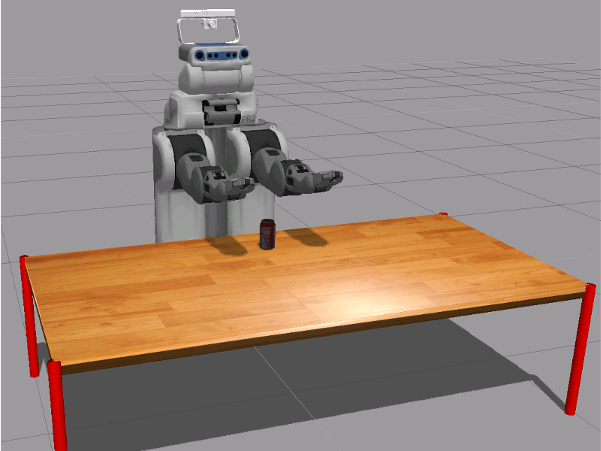### Connect to ROS Network from MATLAB®

In your MATLAB instance on the host computer, run the following commands to initialize ROS global node in MATLAB and connect to the ROS master in the virtual machine through its IP address `ipaddress`. Replace '192.168.233.133' with the IP address of your virtual machine. Specify the port if needed.

```ipaddress = '192.168.116.161'; rosinit(ipaddress,11311);```
```Initializing global node /matlab_global_node_03004 with NodeURI http://192.168.116.1:64988/ ```

### Create Action Clients for Robot Arms and Send Commands

In this task, you send joint trajectories to the PR2 arms. The arms can be controlled via ROS actions. Joint trajectories are manually specified for each arm and sent as separate goal messages via separate action clients.

Create a ROS simple action client to send goal messages to move the right arm of the robot. `rosactionclient` (ROS Toolbox) object and goal message are returned. Wait for the client to connect to the ROS action server.

```[rArm, rGoalMsg] = rosactionclient('r_arm_controller/joint_trajectory_action'); waitForServer(rArm);```

The goal message is a `trajectory_msgs/JointTrajectoryPoint` message. Each trajectory point should specify positions and velocities of the joints.

`disp(rGoalMsg)`
``` ROS JointTrajectoryGoal message with properties: MessageType: 'pr2_controllers_msgs/JointTrajectoryGoal' Trajectory: [1×1 JointTrajectory] Use showdetails to show the contents of the message ```
`disp(rGoalMsg.Trajectory)`
``` ROS JointTrajectory message with properties: MessageType: 'trajectory_msgs/JointTrajectory' Header: [1×1 Header] Points: [0×1 JointTrajectoryPoint] JointNames: {0×1 cell} Use showdetails to show the contents of the message ```

Set the joint names to match the PR2 robot joint names. Note that there are 7 joints to control. To find what joints are in PR2 right arm, type this command in the virtual machine terminal:

```rosparam get /r_arm_controller/joints ```
```rGoalMsg.Trajectory.JointNames = {'r_shoulder_pan_joint', ... 'r_shoulder_lift_joint', ... 'r_upper_arm_roll_joint', ... 'r_elbow_flex_joint',... 'r_forearm_roll_joint',... 'r_wrist_flex_joint',... 'r_wrist_roll_joint'};```

Create setpoints in the arm joint trajectory by creating ROS `trajectory_msgs/JointTrajectoryPoint` messages and specifying the position and velocity of all 7 joints as a vector. Specify a time from the start as a ROS duration object.

```% Point 1 tjPoint1 = rosmessage('trajectory_msgs/JointTrajectoryPoint'); tjPoint1.Positions = zeros(1,7); tjPoint1.Velocities = zeros(1,7); tjPoint1.TimeFromStart = rosduration(1.0); % Point 2 tjPoint2 = rosmessage('trajectory_msgs/JointTrajectoryPoint'); tjPoint2.Positions = [-1.0 0.2 0.1 -1.2 -1.5 -0.3 -0.5]; tjPoint2.Velocities = zeros(1,7); tjPoint2.TimeFromStart = rosduration(2.0);```

Create an object array with the points in the trajectory and assign it to the goal message. Send the goal using the action client. The `sendGoalAndWait` (ROS Toolbox) function will block execution until the PR2 arm finishes executing the trajectory

```rGoalMsg.Trajectory.Points = [tjPoint1,tjPoint2]; sendGoalAndWait(rArm,rGoalMsg);```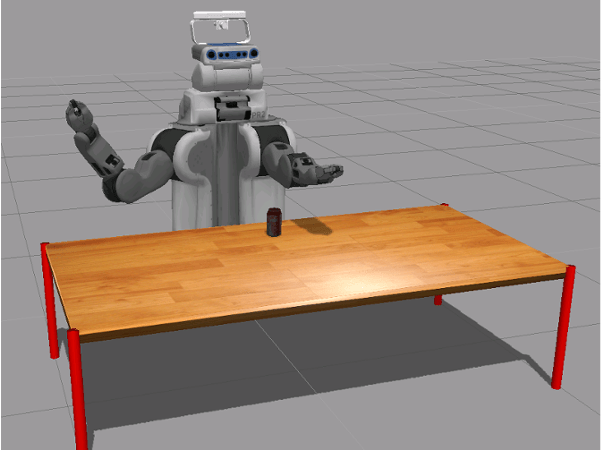Create another action client to send commands to the left arm. Specify the joint names of the PR2 robot.

```[lArm, lGoalMsg] = rosactionclient('l_arm_controller/joint_trajectory_action'); waitForServer(lArm); lGoalMsg.Trajectory.JointNames = {'l_shoulder_pan_joint', ... 'l_shoulder_lift_joint', ... 'l_upper_arm_roll_joint', ... 'l_elbow_flex_joint',... 'l_forearm_roll_joint',... 'l_wrist_flex_joint',... 'l_wrist_roll_joint'};```

Set a trajectory point for the left arm. Assign it to the goal message and send the goal.

```tjPoint3 = rosmessage('trajectory_msgs/JointTrajectoryPoint'); tjPoint3.Positions = [1.0 0.2 -0.1 -1.2 1.5 -0.3 0.5]; tjPoint3.Velocities = zeros(1,7); tjPoint3.TimeFromStart = rosduration(2.0); lGoalMsg.Trajectory.Points = tjPoint3; sendGoalAndWait(lArm,lGoalMsg);```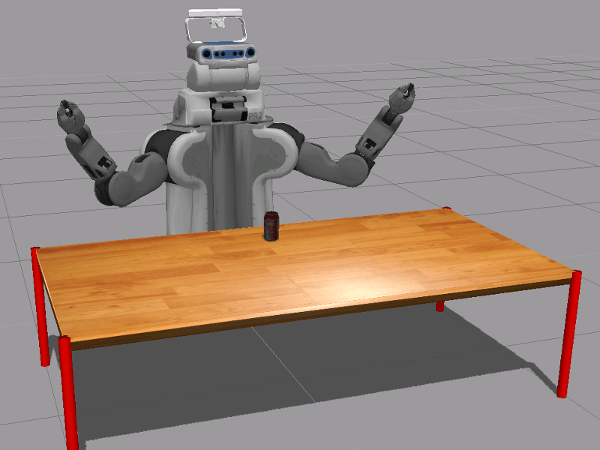### Calculate Inverse Kinematics for an End-Effector Position

In this section, you calculate a trajectory for joints based on the desired end-effector (PR2 right gripper) positions. The `inverseKinematics` class calculates all the required joint positions, which can be sent as a trajectory goal message via the action client. A `rigidBodyTree` object is used to define the robot parameters, generate configurations, and visualize the robot in MATLAB®.

Perform The following steps:

• Get the current state of the PR2 robot from the ROS network and assign it to a `rigidBodyTree` object to work with the robot in MATLAB®.

• Define an end-effector goal pose.

• Visualize the robot configuration using these joint positions to ensure a proper solution.

• Use inverse kinematics to calculate joint positions from goal end-effector poses.

• Send the trajectory of joint positions to the ROS action server to command the actual PR2 robot.

### Create a Rigid Body Tree in MATLAB®

Load a PR2 robot as a `rigidBodyTree` object. This object defines all the kinematic parameters (including joint limits) of the robot.

`pr2 = exampleHelperWGPR2Kinect;`

### Get the Current Robot State

Create a subscriber to get joint states from the robot.

`jointSub = rossubscriber('joint_states');`

Get the current joint state message.

`jntState = receive(jointSub);`

Assign the joint positions from the joint states message to the fields of a configuration struct that the `pr2` object understands.

`jntPos = exampleHelperJointMsgToStruct(pr2,jntState);`

### Visualize the Current Robot Configuration

Use `show` to visualize the robot with the given joint position vector. This should match the current state of the robot.

`show(pr2,jntPos)`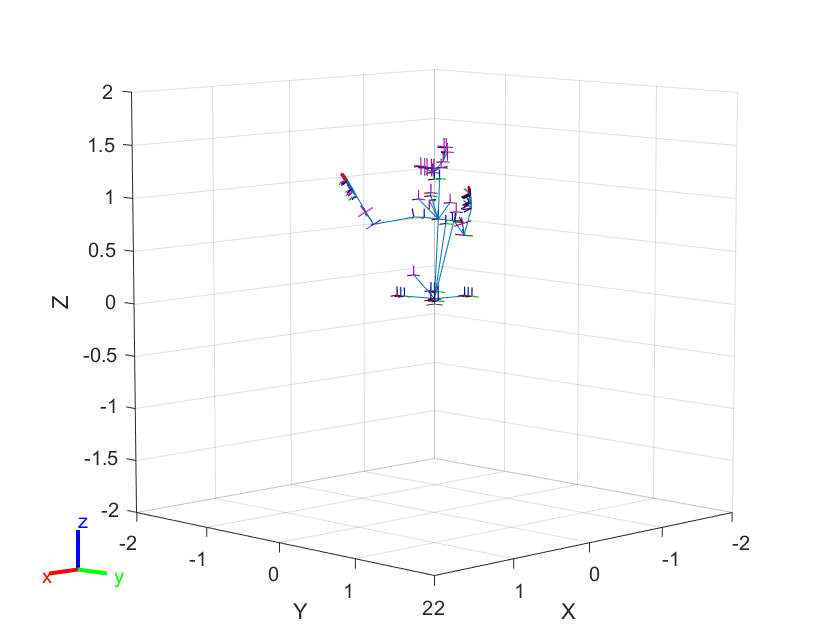```ans = Axes (Primary) with properties: XLim: [-2 2] YLim: [-2 2] XScale: 'linear' YScale: 'linear' GridLineStyle: '-' Position: [0.1300 0.1100 0.7750 0.8150] Units: 'normalized' Show all properties ```

Create an `inverseKinematics` object from the `pr2` robot object. The goal of inverse kinematics is to calculate the joint angles for the PR2 arm that places the gripper (i.e. the end-effector) in a desired pose. A sequence of end-effector poses over a period of time is called a trajectory.

In this example, we will only be controlling the robot's arms. Therefore, we place tight limits on the torso-lift joint during planning.

```torsoJoint = pr2.getBody('torso_lift_link').Joint; idx = strcmp({jntPos.JointName}, torsoJoint.Name); torsoJoint.HomePosition = jntPos(idx).JointPosition; torsoJoint.PositionLimits = jntPos(idx).JointPosition + [-1e-3,1e-3];```

Create the `inverseKinematics` object.

`ik = inverseKinematics('RigidBodyTree', pr2);`

Disable random restart to ensure consistent IK solutions.

`ik.SolverParameters.AllowRandomRestart = false;`

Specify weights for the tolerances on each component of the goal pose.

```weights = [0.25 0.25 0.25 1 1 1]; initialGuess = jntPos; % current jnt pos as initial guess```

Specify end-effector poses related to the task. In this example, PR2 will reach to the can on the table, grasp the can, move it to a different location and set it down again. We will use the `inverseKinematics` object to plan motions that smoothly transition from one end-effector pose to another.

Specify the name of the end effector.

`endEffectorName = 'r_gripper_tool_frame';`

Specify the can's initial (current) pose and the desired final pose.

```TCanInitial = trvec2tform([0.7, 0.0, 0.55]); TCanFinal = trvec2tform([0.6, -0.5, 0.55]);```

Define the desired relative transform between the end-effector and the can when grasping.

`TGraspToCan = trvec2tform([0,0,0.08])*eul2tform([pi/8,0,-pi]);`

Define the key waypoints of a desired Cartesian trajectory. The can should move along this trajectory. The trajectory can be broken up as follows:

• Open the gripper

• Move the end-effector from current pose to `T1` so that the robot will feel comfortable to initiate the grasp

• Move the end-effector from `T1` to `TGrasp` (ready to grasp)

• Close the gripper and grab the can

• Move the end-effector from `TGrasp` to `T2` (lift can in the air)

• Move the end-effector from `T2` to `T3` (move can levelly)

• Move the end-effector from `T3` to `TRelease` (lower can to table surface and ready to release)

• Open the gripper and let go of the can

• Move the end-effector from `TRelease` to `T4` (retract arm)

```TGrasp = TCanInitial*TGraspToCan; % The desired end-effector pose when grasping the can T1 = TGrasp*trvec2tform([0.,0,-0.1]); T2 = TGrasp*trvec2tform([0,0,-0.2]); T3 = TCanFinal*TGraspToCan*trvec2tform([0,0,-0.2]); TRelease = TCanFinal*TGraspToCan; % The desired end-effector pose when releasing the can T4 = TRelease*trvec2tform([-0.1,0.05,0]);```

The actual motion will be specified by `numWaypoints` joint positions in a sequence and sent to the robot through the ROS simple action client. These configurations will be chosen using the `inverseKinematics` object such that the end effector pose is interpolated from the initial pose to the final pose.

### Execute the Motion

Specify the sequence of motions.

`motionTask = {'Release', T1, TGrasp, 'Grasp', T2, T3, TRelease, 'Release', T4};`

Execute each task specified in `motionTask` one by one. Send commands using the specified helper functions.

```for i = 1: length(motionTask) if strcmp(motionTask{i}, 'Grasp') exampleHelperSendPR2GripperCommand('right',0.0,1000,false); continue end if strcmp(motionTask{i}, 'Release') exampleHelperSendPR2GripperCommand('right',0.1,-1,true); continue end Tf = motionTask{i}; % Get current joint state jntState = receive(jointSub); jntPos = exampleHelperJointMsgToStruct(pr2, jntState); T0 = getTransform(pr2, jntPos, endEffectorName); % Interpolating between key waypoints numWaypoints = 10; [s, sd, sdd, tvec] = trapveltraj([0 1], numWaypoints, 'AccelTime', 0.4); % Relatively slow ramp-up to top speed TWaypoints = transformtraj(T0, Tf, [0 1], tvec, 'TimeScaling', [s; sd; sdd]); % end-effector pose waypoints jntPosWaypoints = repmat(initialGuess, numWaypoints, 1); % joint position waypoints rArmJointNames = rGoalMsg.Trajectory.JointNames; rArmJntPosWaypoints = zeros(numWaypoints, numel(rArmJointNames)); % Calculate joint position for each end-effector pose waypoint using IK for k = 1:numWaypoints jntPos = ik(endEffectorName, TWaypoints(:,:,k), weights, initialGuess); jntPosWaypoints(k, :) = jntPos; initialGuess = jntPos; % Extract right arm joint positions from jntPos rArmJointPos = zeros(size(rArmJointNames)); for n = 1:length(rArmJointNames) rn = rArmJointNames{n}; idx = strcmp({jntPos.JointName}, rn); rArmJointPos(n) = jntPos(idx).JointPosition; end rArmJntPosWaypoints(k,:) = rArmJointPos'; end % Time points corresponding to each waypoint timePoints = linspace(0,3,numWaypoints); % Estimate joint velocity trajectory numerically h = diff(timePoints); h = h(1); jntTrajectoryPoints = arrayfun(@(~) rosmessage('trajectory_msgs/JointTrajectoryPoint'), zeros(1,numWaypoints)); [~, rArmJntVelWaypoints] = gradient(rArmJntPosWaypoints, h); for m = 1:numWaypoints jntTrajectoryPoints(m).Positions = rArmJntPosWaypoints(m,:); jntTrajectoryPoints(m).Velocities = rArmJntVelWaypoints(m,:); jntTrajectoryPoints(m).TimeFromStart = rosduration(timePoints(m)); end % Visualize robot motion and end-effector trajectory in MATLAB(R) hold on for j = 1:numWaypoints show(pr2, jntPosWaypoints(j,:),'PreservePlot', false); exampleHelperShowEndEffectorPos(TWaypoints(:,:,j)); drawnow; pause(0.1); end % Send the right arm trajectory to the robot rGoalMsg.Trajectory.Points = jntTrajectoryPoints; sendGoalAndWait(rArm, rGoalMsg); end```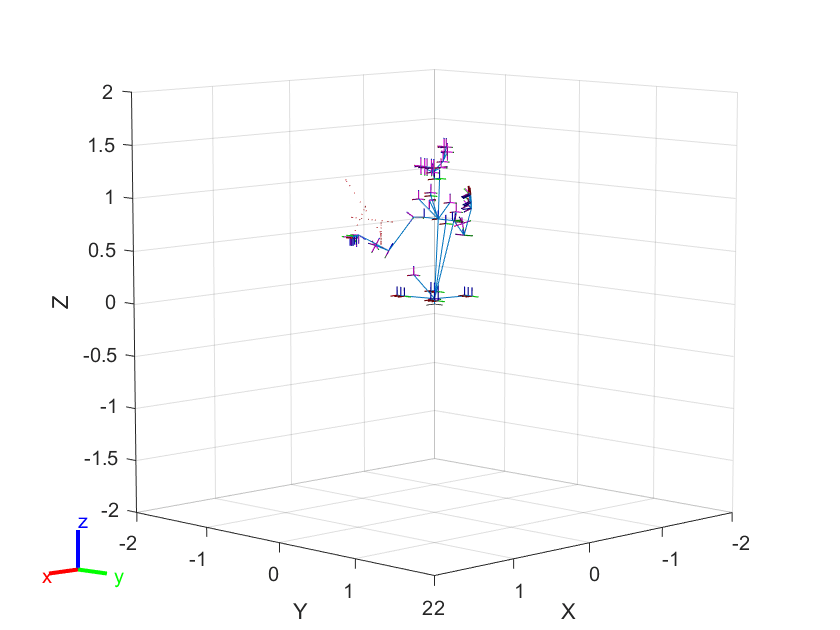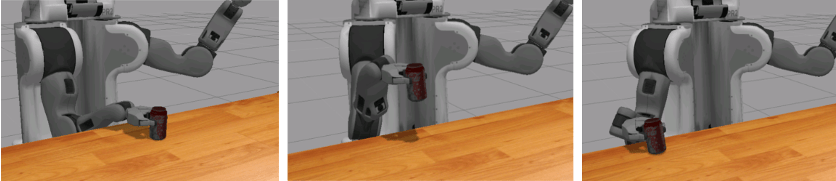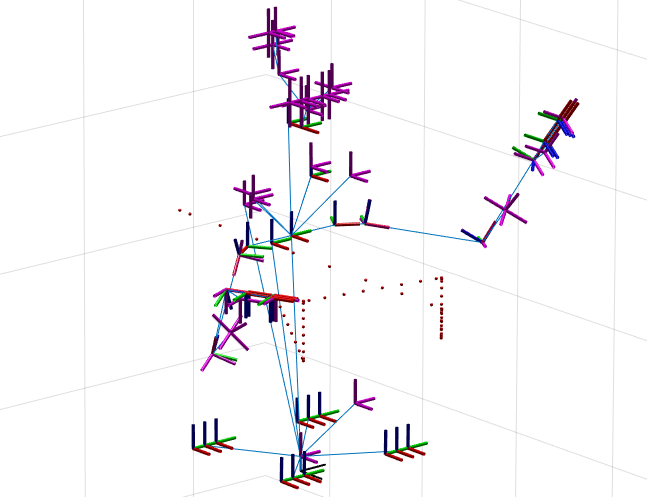### Wrap Up

Move arm back to starting position.

```exampleHelperSendPR2GripperCommand('r',0.0,-1) rGoalMsg.Trajectory.Points = tjPoint2; sendGoal(rArm, rGoalMsg);```

Call `rosshutdown` to shutdown ROS network and disconnect from the robot.

`rosshutdown`
```Shutting down global node /matlab_global_node_03004 with NodeURI http://192.168.116.1:64988/ ```